# Power Analysis

### Details and examples of other methods are accessible via the menu bar on top of the page and in the online manual of all functions.

library(PowerTOST) # attach the library

# Defaults

Note that analysis of untransformed data (logscale = FALSE) is not supported. The terminology of the design argument follows this pattern: treatments x sequences x periods.

With x <- pa.ABE(...), x <- pa.scABE(...), and x <- pa.NTIDFA(...) results are given as an S3 object 1 which can be printed, plotted, or both.

The estimated sample sizes give always the total number of subjects (not subject/sequence in crossovers or subjects/group in a parallel design – like in some other software packages).

## Function pa.ABE()

Parameter Argument Purpose Default
CV CV CV none
$$\small{\theta_0}$$ theta0 ‘True’ or assumed deviation of T from R 0.95
$$\small{\pi}$$ targetpower Minimum desired power 0.80
$$\small{\pi}$$ minpower Minimum acceptable power 0.70
design design Planned design "2x2x2"
passed ... Arguments to power.TOST() none

If no additional arguments are passed, the defaults of power.TOST() are applied, namely alpha = 0.05, theta1 = 0.80, theta2 = 1.25.

Arguments targetpower, minpower, theta0, theta1, theta2, and CV have to be given as fractions, not in percent.
CV is generally the within- (intra-) subject coefficient of variation. In replicate designs only homoscedasticity (CVwT = CVwR) is supported. For design = "parallel" it is the total (a.k.a. pooled) CV.

The conventional TR|RT (a.k.a. AB|BA) design can be abbreviated as "2x2". Some call the "parallel" design a ‘one-sequence’ design. The "paired" design has two periods but no sequences, e.g., in studying linear pharmacokinetics a single dose is followed by multiple doses. A profile in steady state (T) is compared to the one after the single dose (R). Note that the underlying model assumes no period effects.

## Function pa.scABE()

Parameter Argument Purpose Default
CV CV CV none
$$\small{\theta_0}$$ theta0 ‘True’ or assumed deviation of T from R 0.90
$$\small{\pi}$$ targetpower Minimum desired power 0.80
$$\small{\pi}$$ minpower Minimum acceptable power 0.70
design design Planned replicate design "2x2x3"
regulator regulator ‘target’ jurisdiction (see below) "EMA"
nsims nsims Number of simulations 1e5
passed ... Arguments to power.scABEL() or power.RSABE() none

If no additional arguments are passed, the defaults of power.scABEL() and power.RSABE() are applied, namely alpha = 0.05, theta1 = 0.80, theta2 = 1.25. Note the recommended 2 default $$\small{\theta_0}$$ 0.90 for HVDPs.
regulator can be "EMA", "HC", or "FDA".

Arguments targetpower, minpower, theta0, theta1, theta2, and CV have to be given as fractions, not in percent. CV is the within- (intra-) subject coefficient of variation, where only homoscedasticity (CVwT = CVwR) is supported.

## Function pa.NTIDFDA()

Parameter Argument Purpose Default
CV CV CV none
$$\small{\theta_0}$$ theta0 ‘True’ or assumed deviation of T from R 0.975
$$\small{\pi}$$ targetpower Minimum desired power 0.80
$$\small{\pi}$$ minpower Minimum acceptable power 0.70
design design Planned replicate design "2x2x4"
nsims nsims Number of simulations 1e5
passed ... Arguments to power.NTIDFDA() none

If no additional arguments are passed, the defaults of power.NTIDFDA() are applied, namely alpha = 0.05, theta1 = 0.80, theta2 = 1.25. Note the default $$\small{\theta_0}$$ 0.975 for NTIDs since the FDA requires tighter batch release limits of ±5% for them.

Arguments targetpower, minpower, theta0, theta1, theta2, and CV have to be given as fractions, not in percent. CV is the within- (intra-) subject coefficient of variation, where only homoscedasticity (CVwT = CVwR) is supported.

# Examples

## Average Bioequivalence

##### Example 1

Example 3 of vignette ABE. Assumed CV 0.20, $$\small{\theta_0}$$ 0.92.

pa.ABE(CV = 0.20, theta0 = 0.92)
# Sample size plan ABE
#  Design alpha  CV theta0 theta1 theta2 Sample size Achieved power
#     2x2  0.05 0.2   0.92    0.8   1.25          28       0.822742
#
# Power analysis
# CV, theta0 and number of subjects which lead to min. acceptable power of at least 0.7:
#  CV= 0.2377, theta0= 0.9001
#  n = 21 (power= 0.7104)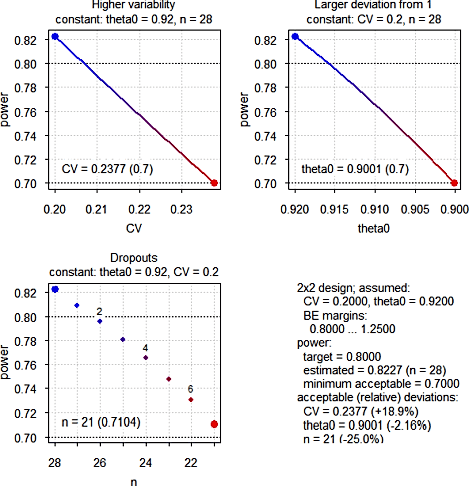Fig. 1 Power Analysis (in each panel one argument is varied and others kept constant)

The most critical parameter is $$\small{\theta_0}$$, whereas dropouts are the least important. We will see a similar pattern in other approaches as well.

## Reference-scaled Average Bioequivalence

### Highly Variable Drugs / Drug Products

#### EMA

##### Example 2

Assumed intra-subject CV 0.55.

pa.scABE(CV = 0.55)
# Sample size plan scABE (EMA/ABEL)
#  Design alpha CVwT CVwR theta0 theta1 theta2 Sample size Achieved power
#   2x3x3  0.05 0.55 0.55    0.9    0.8   1.25          42        0.80848
#  Target power
#           0.8
#
# Power analysis
# CV, theta0 and number of subjects which lead to min. acceptable power of at least 0.7:
#  CV= 0.6668, theta0= 0.8689
#  n = 33 (power= 0.7085)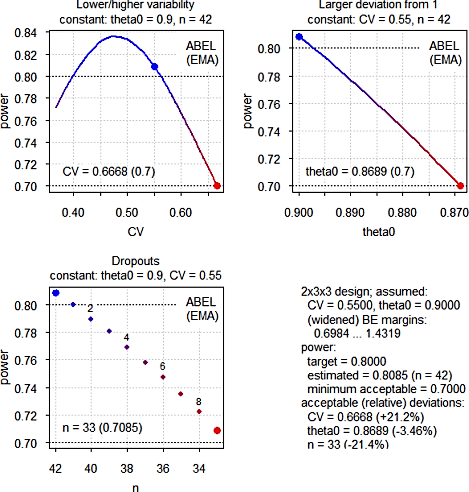Fig. 2 Power Analysis (in each panel one argument is varied and others kept constant)

The idea behind reference-scaling is to preserve power even for high variability without requiring extreme sample sizes. However, we make two interesting observations. At CVwR 0.55 already the upper cap of scaling (50%) cuts in and the expanded limits are the same as at CVwR 0.50. Therefore, if the variability increases, power decreases. On the other hand, if the CV decreases, power increases first (because being affected by the upper cap is less likely) and then decreases again (because the limits can be less expanded).

##### Example 3

Assumed intra-subject CV 0.40, 4-period full replicate design.

pa.scABE(CV = 0.40, design = "2x2x4")
# Sample size plan scABE (EMA/ABEL)
#  Design alpha CVwT CVwR theta0 theta1 theta2 Sample size Achieved power
#   2x2x4  0.05  0.4  0.4    0.9    0.8   1.25          30        0.80656
#  Target power
#           0.8
#
# Power analysis
# CV, theta0 and number of subjects which lead to min. acceptable power of at least 0.7:
#  CV= 0.6938, theta0= 0.8763
#  n = 23 (power= 0.7123)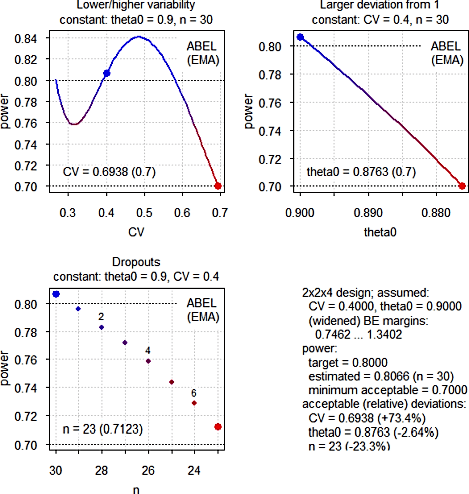Fig. 3 Power Analysis (in each panel one argument is varied and others kept constant)

Here we see a different pattern. With increasing variability power increases (due to more expanding) up to the cap of scaling where it starts to decrease like in the previous example. If the variability decreases, power decreases as well (less expanding). However, close the the switching CVwR (30%) power increases again. Although we cannot scale anymore, with 30 subjects the study is essentially ‘overpowered’ for ABE.

##### Example 4

Same assumptions (CV, $$\small{\theta_0}$$) like in Example 2.

pa.scABE(CV = 0.55, regulator = "HC")
# Sample size plan scABE (HC/ABEL2)
#  Design alpha CVwT CVwR theta0 theta1 theta2 Sample size Achieved power
#   2x3x3  0.05 0.55 0.55    0.9    0.8   1.25          39        0.81418
#  Target power
#           0.8
#
# Power analysis
# CV, theta0 and number of subjects which lead to min. acceptable power of at least 0.7:
#  CV= 0.7599, theta0= 0.8647
#  n = 30 (power= 0.7125)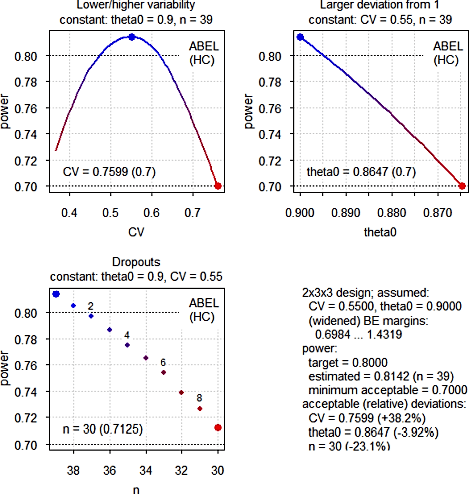Fig. 4 Power Analysis (in each panel one argument is varied and others kept constant)

Since we are close to Health Canada’s upper cap of 57.4%, power decreases on both sides. Note that three subjects less than for the EMA’s method are required and CVwR can increase to ~0.76 until we reach the minimum acceptable power – which is substantially higher than the ~0.67 for the EMA.

#### FDA

##### Example 5

Same assumptions (CV, $$\small{\theta_0}$$) like in Example 2 and Example 4.

pa.scABE(CV = 0.55, regulator = "FDA")
# Sample size plan scABE (FDA/RSABE)
#  Design alpha CVwT CVwR theta0 theta1 theta2 Sample size Achieved power
#   2x3x3  0.05 0.55 0.55    0.9    0.8   1.25          30        0.80034
#  Target power
#           0.8
#
# Power analysis
# CV, theta0 and number of subjects which lead to min. acceptable power of at least 0.7:
#  CV= 0.9713, theta0= 0.8652
#  n = 23 (power= 0.7001)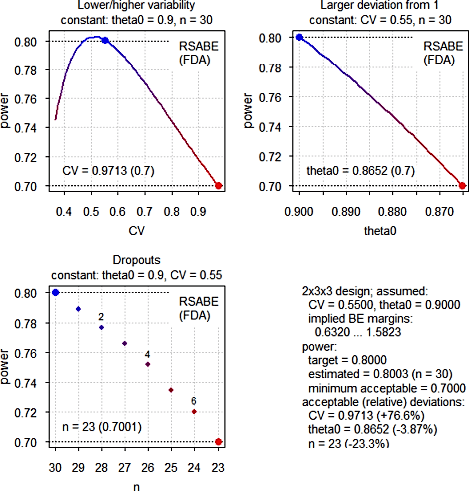Fig. 5 Power Analysis (in each panel one argument is varied and others kept constant)

A similar pattern like the one of Health Canada, although due to the different regulatory constants ($$\small{\theta_s\approx0.8926}$$ vs $$\small{k=0.760}$$ for Health Canada and the EMA) nine subjects less (and twelve less than for the EMA) are required. Due to unlimited scaling the CV can increase more.

### Narrow Therapeutic Index Drugs (FDA)

##### Example 6

Assumed intra-subject CV 0.125.

pa.NTIDFDA(CV = 0.125)
# Sample size plan RSABE NTID
#  Design alpha  CVwT  CVwR theta0 theta1 theta2 Sample size Achieved power
#   2x2x4  0.05 0.125 0.125  0.975    0.8   1.25          16        0.82278
#  Target power
#           0.8
#
# Power analysis
# CV, theta0 and number of subjects which lead to min. acceptable power of at least 0.7:
#  CV = (0.0716, 0.3059), theta0= 0.9569
#  n = 13 (power= 0.7064)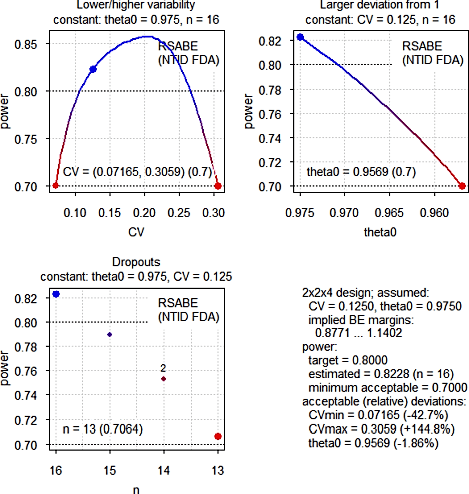Fig. 6 Power Analysis (in each panel one argument is varied and others kept constant)

With decreasing variability power decreases because the scaled limits become narrower. With increasing variability we gain power because we have to scale less until at ~21.4% the additional criterion ‘must pass conventional BE limits of 80.00–125.00%’ cuts in.

# Cave!

The power analysis is  not  a substitute for the ‘Sensitivity Analysis’ recommended by the ICH. 3 In a real study a combination of all effects occurs simultaneously. It is up to you to decide on reasonable combinations and analyze their respective power.

# Authors

function author(s)
pa.ABE, pa.scABE Idea and original code by Helmut Schütz with modifications by Detlew Labes
pa.NTIDFDA Detlew Labes acc. to code by Helmut Schütz for pa.ABE and pa.scABE